# Vocabulary Geometry Flashcards

Set Details Share
created 13 years ago by fmarlatt
12,996 views
These terms compliment the Pearson enVision math series for fourth grade
updated 11 years ago by fmarlatt
Page to share:
Embed this setcancel
COPY
code changes based on your size selection
Size:
X

1

point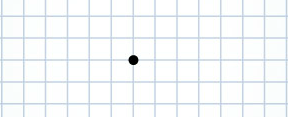an exact location in space

2

acute angle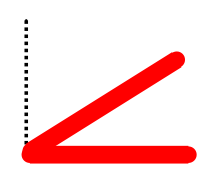an angle that is less than 90 degrees and smaller than a right angle

3

Hexagon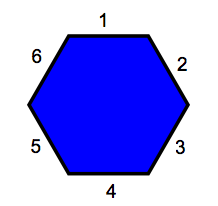a polygon with 6 sides and 6 vertices

4

intersecting lines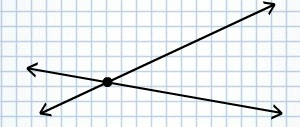the 2 line segments cross each other at a point

5

parallel lines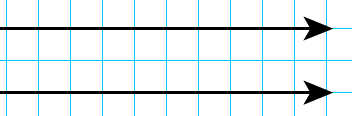lines that are equal distance apart at every point and they never intersect
The strips on the monarch caterpillar are parallel.

6

symmetric
symmetrical
lines of symmetry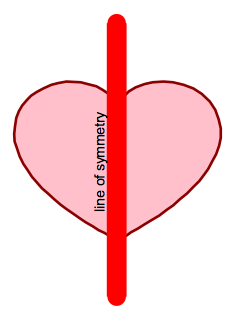a figure is symmetric if it can be folded into 2 congruent halves that fit on top of each other. The line of symmetry divides the valentine heart into 2 congruent shapes.

7

line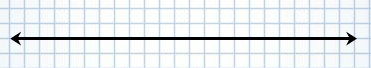a straight path of points that goes on forever in both directions

8

plane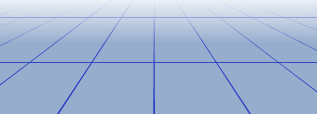an endless flat surface

9

perpendicular lines2 lines that intersect at a right angle

10

line segment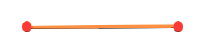part of a line that has 2 end points

11

ray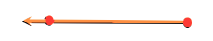part of a line that has 1 end point and extends forever in one direction

12

angle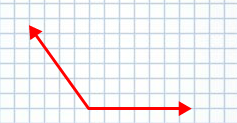a figure formed by 2 rays that have the same endpoint

13

right angle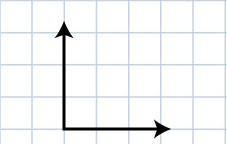an angle that measures exactly 90º

14

obtuse angle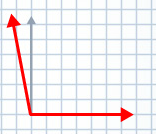and angle that measures more than 90º

15

straight angle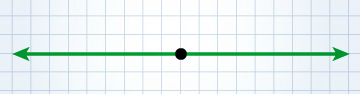an angle that measures exactly 180º

16

polygon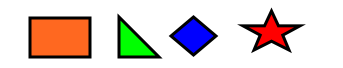a closed shape made up of line segments

17

side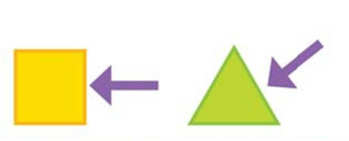each of the line segments of a polygon

18

vertex, vertices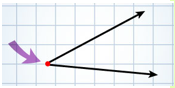the point where 2 rays meet to form an angle. the points where the sides of a polygon meet.

19

triangle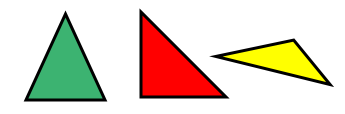a polygon with 3 sides and 3 angles

20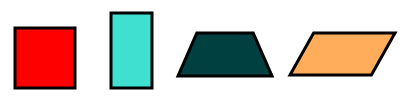a polygon with 4 sides and 4 angles

21

pentagon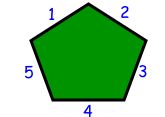a polygon with 5 sides and 5 angles

22

octagon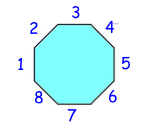a polygon with 8 sides and 8 angles

23

parallelogram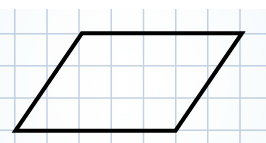a quadrilateral in which the opposite sides are parallel

24

rectangle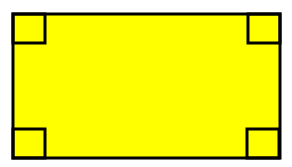a parallelogram with 4 right angles

25

square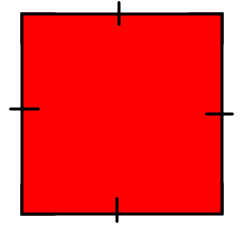a parallelogram with 4 right angles and all sides the same length

26

rhombus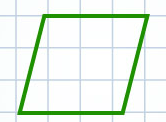a parallelogram with all sides the same length

27

trapezoid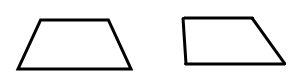a quadrilateral with only 1 pair of opposite sides that are parallel

28

equilateral triangle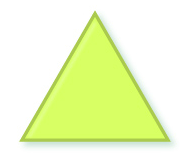a triangle with 3 equal sides

29

isosceles triangle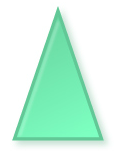a triangle that has 2 equal sides

30

scalene triangle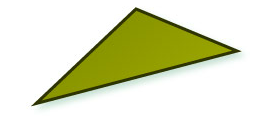a triangle that has no sides that as the same length

31

right triangle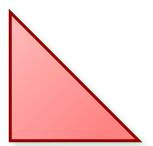a triangle with 1 right angle

32

acute triangle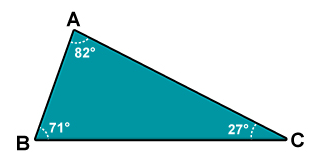a triangle with 3 acute angles

33

obtuse triangle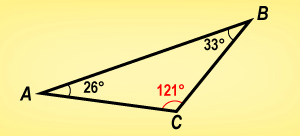a triangle with one obtuse angle

34

congruent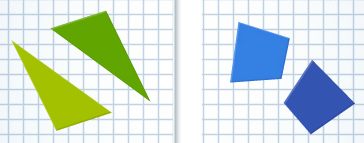2 shapes that are the same shape and the same size

35

similar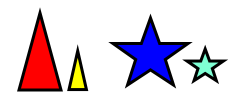2 shapes that are the same shape but not the same size

36

classify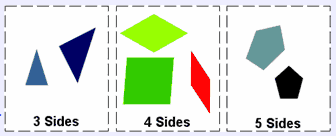To arrange in groups, by some property.

These shapes are classified by the number of sides.

37

ellipse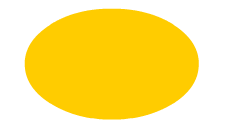a 2 dimensional shape that resembles a flatten circle
An ellipse has only 2 lines of symmetry

38

equiangular39

two dimensional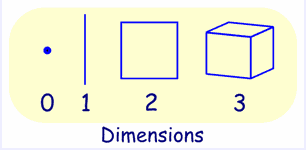a shape that has height and width but no depth, Squares, Circles, Triangles, etc are two dimensional objects

40

congruent polygons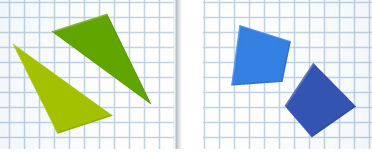2 polygons that are the same shape and same size